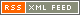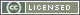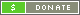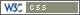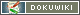# Link margin calculations (line of sight)

Knowing antenna gain, receiver sensitivity and transmit power, calculate the Link Margin

### Calculate the Path Loss in dB

Path Loss (dB) = 37 dB + 20log(f) + 20log(d) with f in MHz and d in miles.

### Calculate the maximum Received Signal Strength in dBm

Rss(dBm) = Transmit Power(dBm) + Receive Antenna Gain(dB) + transmit antenna gain(dB) – path loss(dB)

### Estimate Fade Margin in dB

Outside operation with no obstruction, half wave antenna protruding from electronic enclosure: Fade Margin should be at least 5dB Inside building operation or compromised antenna (inside enclosure, antenna less than ½ wavelength from other conductors or operator's body: Fade Margin should be at least 40dB